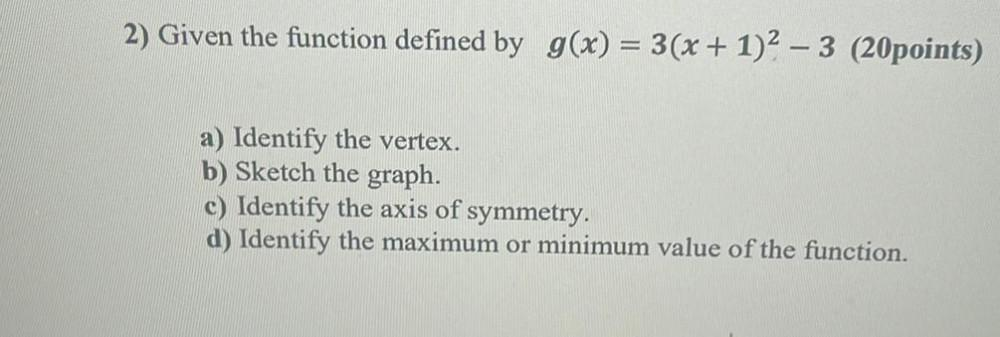Question:

# 2) Given the function defined by g(x) = 3(x + 1)2 – 3 (20points) a) Identify the vertex. b) Sketch the graph. c) Identify the ax2) Given the function defined by g(x) = 3(x + 1)2 – 3 (20points) a) Identify the vertex. b) Sketch the graph. c) Identify the axis of symmetry. d) Identify the maximum or minimum value of the function.